Next: Quantum Effects on Classical Integrals Up: Chapter 11 Previous: Choice of Basis Vectors

# Replacement of Sums over All States with Sums over Eigenstates

Sums over States

Lectures 1-6 presented a demonstration of the powers of classical statistical mechanics. Our calculations were based on the use of ensembles, an ensemble being a list of all possible states of the system, together with the statistical weight assigned to each state. The list of allowed states and statistical weights is often given implicitly by listing a series of constraints: for the canonical ensemble, the constraints are fixed N, V, T. For the 1-atom ideal gas, the states of the system are specified by giving the position and momentum of the gas molecule. The particle positionis allowed to lie anywhere within V, while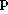may have any vector value.

The treatment leading to eq. 11.6 masked a fundamental difference between the sums used used in Lectures 8 and 9 and the sums used in all previous Lectures. In the earlier Lectures, the ensemble sum was taken over all states whatsoever of the system. In Lectures 8 and 9, the sum was taken over the system's energy eigenstates. These energy eigenstates are a complete set of states. However, in quantum mechanics, the adjective complete assumes a specific technical meaning, namely that is a set of states is complete if an arbitrary state of the system can written as a sum over the complete set of states. For any system, many allowed states are not included in the complete set of states, namely the mixed states, which are are linear combinations of the complete set of basis states. Note the fundamental difference between the classical and quantum calculations. In the classical calculation, the 'complete' set of states included every single state. In the quantum calculation, the 'complete' set of states included some states but not others.

As an example of the distinction between a complete set of state vectors and a complete set of basis vectors, consider the model spin system treated above. A complete set of (basis) states for this system is formed by the energy eigenstatesand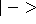. An alternative complete set of (basis) states for the system is composed of the helicity statesand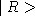. Any other state of the system may be written as a linear combination of the energy eigenstates or as a linear combination of the helicity states. By applying Gramm-Schmidt orthonormalization, any non-zero state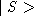whatsoever of the system may be taken as a basis state; the orthogonality requirement then determines the other basis state. This is a two-dimensional system. No matter which (non-zero) state of the system is chosen to be one of the basis vectors, Gramm-Schmidt orthonormalization will yield two and only two basis vectors for this system. Since any single state of the system may be chosen as one of the two basis vectors, there is absolutely no fundamental physical distinction, without reference to the current choice of basis, in which any particular set may be said to be `pure'or `mixed'.

While the spin system has only two basis vectors, it has an infinite number of states. Limiting ourselves to normalized states, the complete list of all states of the system, including pure and mixed states, may be writtenHere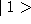and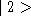are the basis vectors for the system,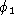andare phase factors (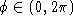), and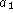and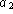are amplitudes, with. There are two basis vectors, but there are a three-fold infinite set of statesof the system. (In Dirac's formalization of quantum mechanics, the direction but not the magnitude ofhas physical significance, so the conditionwould be lifted.)

If the canonical ensemble includes all states of the system, an average over a canonical ensemble for the two-level spin should have a sum over all of the states represented by eq. 11.10, not just a sum (like eqs. 11.5 or 11.9) over a pair of basis states. To write this average over a canonical ensemble, recognize that an arbitrary stateof the system is characterized by the amplitudes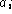and phases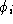of its eigenvector components. The pure states are those in which some oneis unity, while the otherall vanish. The phasesrange freely over the interval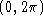. Theare free to have any real value, subject to the restriction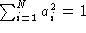. The phase factors absorb the sign of; the amplitudes are restricted to be positive semidefinite. A sum over all states of a quantum system as opposed to a sum over a complete set of states, may be expressed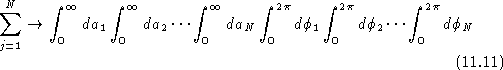so that the quantum ensemble average becomes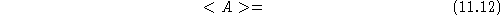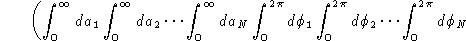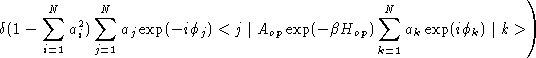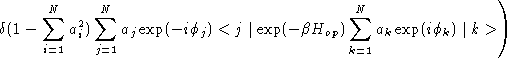Inspection allows some simplification of these integrals. First extract the double sum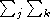to outside the integrals. Each term of the double sum contains a factorNothing outside this factor, e.g., E, should depend onor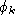. The self terms of the double sum yield factors. The distinct terms of the double sum vanish, reducing the double sum to a single sum, namely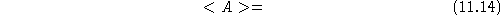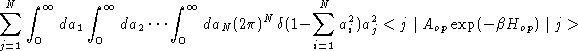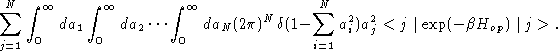The integrals in eq. 11.14 need not be evaluated explicitly. The quantum averagesand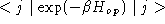depend on J but not on the. Except for the label j, the integralsare the same in every term of the numerator sum and in every term of the denominator sum. Substituting eq. 11.15 into eq. 11.14,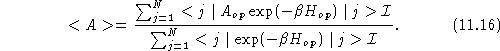While complicated in structure, the factors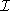above and below are constants, which may be cancelled, giving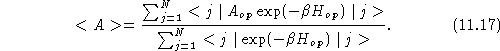for the ensemble average and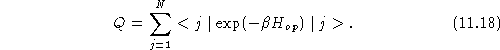for the partition function. An ensemble average over all states of a quantum system thus reduces to an ensemble average over a complete set of basis states. Eq. 11.18 justifies our claim above that the partition function can be written as a sum over energy eigenstates. It is important to emphasize that the results of this section have nothing to do with the results of the previous section. In the previous section, we showed that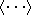is independent of the choice of basis set. In this section, we showed that computingover any choice of basis set gives the same result as computingover all states of the system.Next: Quantum Effects on Classical Integrals Up: Chapter 11 Previous: Choice of Basis Vectors

Nicholas V Sushkin
Sun Jun 30 15:55:07 EDT 1996